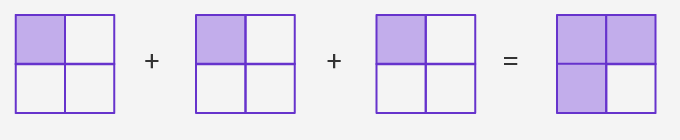Multiplying Fraction By A Whole Number - Definition with Examples

The Complete K-5 Math Learning Program Built for Your Child

• 30 Million Kids

Loved by kids and parent worldwide

• 50,000 Schools

Trusted by teachers across schools

• Comprehensive Curriculum

Aligned to Common Core

Multiplying Fraction by a Whole Number

We know that multiplication is repeated addition. So, multiplying a fraction by a whole number is equivalent to adding the fraction for the whole number of times.

For example:

3 x 14can be shown as,Algebraically this means, 3 x 1 4 = 1 4 + 1 4 + 1 4 = 1 + 1 + 1 4 = 3 4

Consider the product 5 x 2 3 .

This is equivalent to adding 23, 5 times. Since the repeated addition can be done by multiplication, this can be done by multiplying the numerator by 5.

That is   5 x 23 = 5x24 = 103

Another way of looking at it is by considering the whole number 5 as a fraction with denominator 1. To multiply two fractions, multiply the numerators and denominators separately and then write their products as the numerators and denominators respectively.

5 x 23 = 51 + 23 =5x21x3 = 103

Since the product is an improper fraction convert it into a mixed number. Divide 10 by 3. The quotient is 3 and the remainder is 1.

Thus,  103 = 313

This can be clearly identified in the geometrical interpretation as shown:

Example:

Catherine is making a cake for which she needs to use a three-fourths cup of oil. If she decides to make three cakes, what would be the amount of oil required?

For three cakes, the amount of the oil used be 3 times of three-fourths cup of oil.

3 x 34 = 31 x 34  =  3x31x3 = 94

Convert the improper fraction into a mixed number.

9 ÷ 4 = Q 2 R 1

Thus, 94 = 2

Therefore, the new recipe would require 2 and a quarter cups of oil.

 Fun fact:  If the multiplicand is a mixed fraction, first convert the mixed fraction into an improper fraction and then multiply.

Example: 5 x 63

First, convert 6 into an improper fraction.

623 = (6x3)+23  =203

So,  5 x 623 = 5 x 203  =51 x2031003

Now, convert 1003 into a mixed fraction.

120 ÷ 3= Q 33 R 1

Thus, 1003 = 3313

Won Numerous Awards & Honors